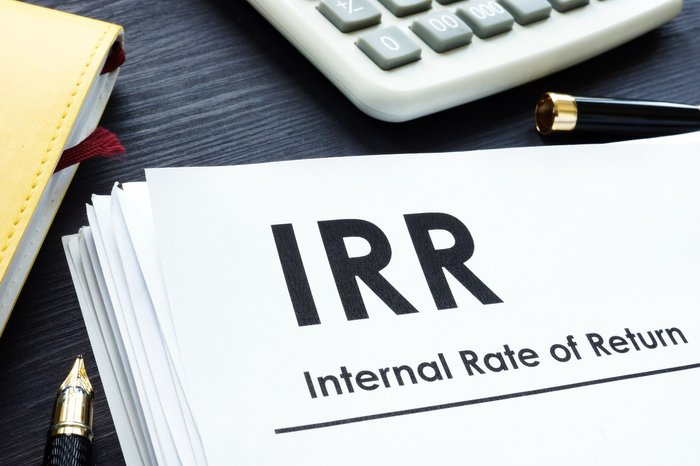Please ensure Javascript is enabled for purposes of website accessibility

# NPV vs. IRR: What's the Difference?

Updated: May 13, 2022, 10:47 a.m.

Whether you are a real estate investor in the midst of deciding where to make a capital investment, or you're working with a capital budget planner on your next big project -- whatever stage you are in will require you to run several analyses to predict the net cash flow of your future endeavor.

Part of being a savvy investor is knowing when to hold them and when to fold them. And you can decide this by calculating the net present value (NPV) and the internal rate of return (IRR). Let's look at both metrics in more detail.Source: Getty images

## Net present value (NPV)

NPV is a metric used to evaluate the present value of the future cash flows a proposed project or investment will generate. NPV is the difference between the present value of cash inflows and the present value of cash outflow. It factors in both inflation and reinvestment rates to provide a precise estimate of a monetary investment's present value against its future value.

We have all heard the cliche that time is money. And that is exactly what the purpose of the NPV calculation is: the evaluation of money over the course of time, the time value of money. To borrow a line from the iconic movie Jerry McGuire, "Show me the money!" -- or for our purposes, show me the NPV!

Note: The time value of money is a principle of finance used to explain how money is worth more in the present than it would be at the same time in the future. This concept is the center of net present value (NPV).

If NPV is a positive value, you can anticipate that there will be positive cash flow and the project will be profitable. This makes this type of project very attractive to investors. On the other hand, if the NPV is negative, you can anticipate the project producing a net loss. If this is the case, you may want to reevaluate your investment.

NPV represents all the future cash flows over the lifespan of a project, making it the central tool used to evaluate the value of an investment based on future cash flows. So, if you have any pending remodel or renovation projects, working with your accountant to help calculate the NPV beforehand will definitely be worth your time and money.

## Internal rate of return (IRR)

As a savvy investor, the IRR is another calculation that you will need to consider before investing in a project. IRR is used to measure the annual rate of return on an investment; its net profitability. The higher the IRR, the more profitable the investment. For this reason, this metric is preferable when attempting to present a proposal to real estate investors.

Individuals or firms who use the IRR method are also doing so with the goal of determining which rate of discount makes the present value of annual cash in flows equal to the original investment.

Unlike NPV, which is expressed in dollars, the IRR is expressed as a percentage. IRR calculates the percentage of the rate of return at which a project's associated cash flows will result in an NPV of zero. It is also the discount rate at which the total present value of costs (expenses) is equivalent to the total present value of the benefits (profits). In layman's terms, IRR tells firms when they will eventually break even.

In addition, IRR tells investors when they will break even. The IRR rule tells investors to take any investment in which the IRR exceeds the cost of capital and leave any investment wherein the cost of capital exceeds the IRR. In short, the higher the IRR, the more money!

To compute IRR, you can use an IRR calculator, but working with your accountant is advisable. Sometimes, a project will require multiple IRR computations, which can be quite tricky. This is the reason computing IRR by hand using the IRR formula is not advisable. Thus, reaching out to an expert is the safest bet.

## NPV vs. IRR: Which is best?

When you are working on capital budgeting , deciding to run an NPV or IRR calculation depends on the project's needs. Typically, NPV is used for long-term projects, and IRR is generally used to tell a corporation how many shares to issue.

So, in the battle of NPV vs.IRR, from an investor’s perspective, IRR will likely win. An investor will most certainly want to know the expected cash in flow and the amount of shares available to purchase. While NPV is used to determine the cumulative value of a potential investment project and may be more useful for the initial investment.

Sometimes, when investors calculate NPV and IRR, the two values conflict. If this situation ever arises, using the NPV method is preferred.

## The bottom line

The bottom line of any investment is to generate a profit. Before taking a leap of faith into any investment, crunching the numbers and calculating the IRR or NPV is advisable. These figures can tell you whether your investment will be profitable. Remember, the more positive the NPV and the higher the IRR, the more green your project could see!

If you need assistance with these calculations, do not go at it alone. Consult with an accountant before starting any new investment.